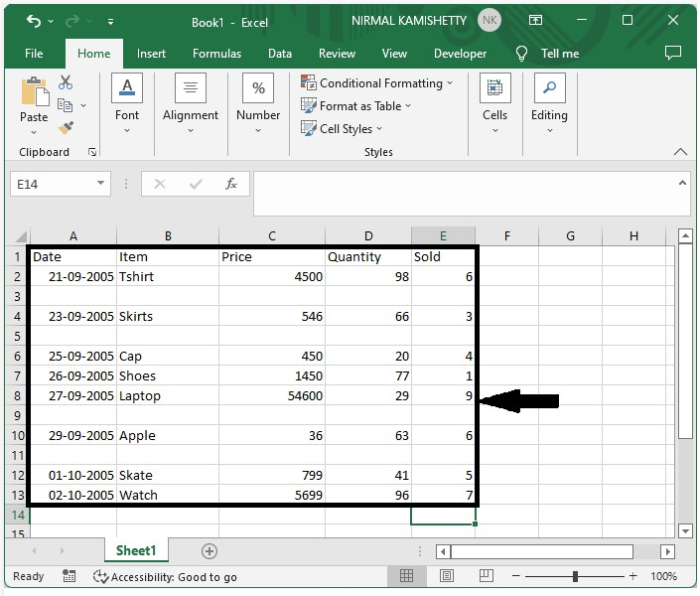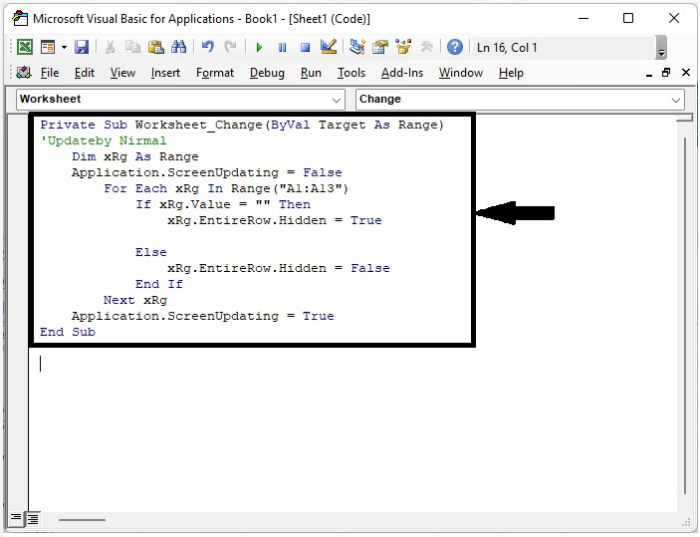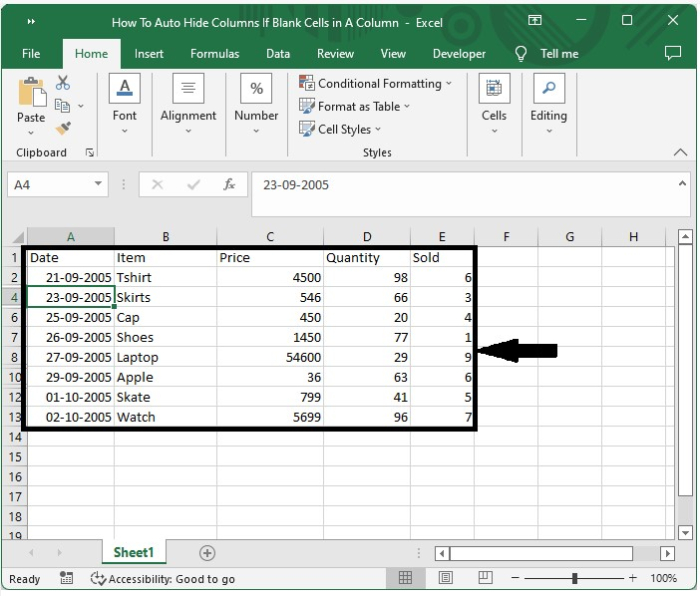# How to Auto-Hide Columns if There are Blank Cells in a Column in Excel?

Sometimes, when we delete data from multiple columns in Excel, we even need to delete the empty columns. But in Excel, deletion of multiple columns at once isn’t possible, and deleting columns individually can take a lot of time. We can solve this problem by hiding the empty columns in Excel.

Read this tutorial to learn how you can automatically hide columns if there are blank cells in a column.

## AutoHide Columns if There are Blank Cells

Here we will first insert VBA code for the sheet, then double-click on any cell to complete our task. Let us see a simple process to understand how we can auto-hide the columns if there are only empty cells in a column in Excel.

Step 1

Let us consider an Excel sheet where the data in the sheet is similar to the data in the below image.Now to solve the problem, right-click on the sheet name and select view code to open the vba application, then type the programme into the text box as shown in the below image.

### Example

Private Sub Worksheet_Change(ByVal Target As Range)
'Updateby Nirmal
Dim xRg As Range
Application.ScreenUpdating = False
For Each xRg In Range("A1:A13")
If xRg.Value = "" Then
xRg.EntireRow.Hidden = True

Else
xRg.EntireRow.Hidden = False
End If
Next xRg
Application.ScreenUpdating = True
End Sub


In the code, A1:A13 is the range you want to apply the code to; we can change the range based on our requirements.Step 2

Now save the sheet as a macro-enabled template and close the VB application using the command Alt + Q. then go back to the Excel sheet and double-click on any empty cell and click on Enter to complete our process, and our final result will be similar to the data shown in the below image.## Conclusion

In this tutorial, we used a simple example to demonstrate how we can auto-hide the columns if there are only empty cells in a column in Excel.

Updated on: 10-Jan-2023

1K+ Views